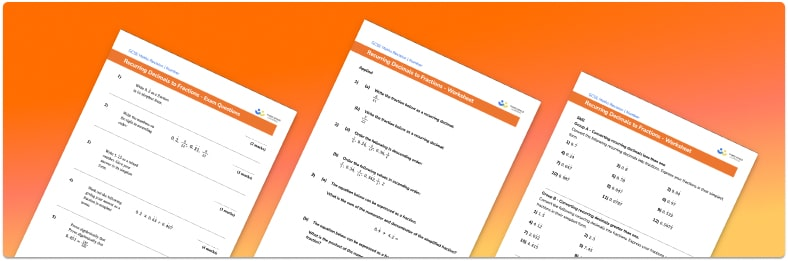# Recurring Decimals To Fractions Worksheet• Section 1 of the recurring decimals to fractions worksheet contains 36 skills-based recurring decimals to fractions questions, in 3 groups to support differentiation
• Section 2 contains 4 applied recurring decimals to fractions questions with a mix of word problems and deeper problem solving questions
• Section 3 contains 5 foundation and higher level GCSE exam style recurring decimals to fractions questions
• Answers and a mark scheme for all recurring decimals to fractions questions are provided
• Questions follow variation theory with plenty of opportunities for students to work independently at their own level
• All questions created by fully qualified expert secondary maths teachers
• Suitable for GCSE maths revision for AQA, OCR and Edexcel exam board

• This field is for validation purposes and should be left unchanged.

You can unsubscribe at any time (each email we send will contain an easy way to unsubscribe). To find out more about how we use your data, see our privacy policy.

### Recurring decimals to fractions at a glance

Recurring decimals, or repeating decimals, are decimal numbers with an indefinite pattern of repeating digits.

To convert recurring decimals to fractions we use a method which involves multiplying the decimal by a power of 10 in such a way that the pattern of numbers after the decimal point lines up with the original decimal. We can then subtract the two numbers, leaving an integer which we can use to write the fraction.

For example, let’s consider the decimal 0.6363636363…, we can call this number x. If we multiply this number by 100 we get 63.63636363…= 100x . We then need to subtract x from 100x to leave we are left with the integer 63 equal to 99x. Finally, dividing both sides by 99 gives us a fraction with 63 as the numerator and 99 as the denominator. So the recurring decimal 0.636363… is equal to 63 over 99. We usually work with fractions in their simplest form so simplifying this fraction we get 711.

Looking forward, students can then progress to additional number worksheets, for example aor aFor more teaching and learning support on Number our GCSE maths lessons provide step by step support for all GCSE maths concepts.

## Do you have KS4 students who need more focused attention to succeed at GCSE?There will be students in your class who require individual attention to help them succeed in their maths GCSEs. In a class of 30, it’s not always easy to provide.

Help your students feel confident with exam-style questions and the strategies they’ll need to answer them correctly with our dedicated GCSE maths revision programme.

Lessons are selected to provide support where each student needs it most, and specially-trained GCSE maths tutors adapt the pitch and pace of each lesson. This ensures a personalised revision programme that raises grades and boosts confidence.

Find out more